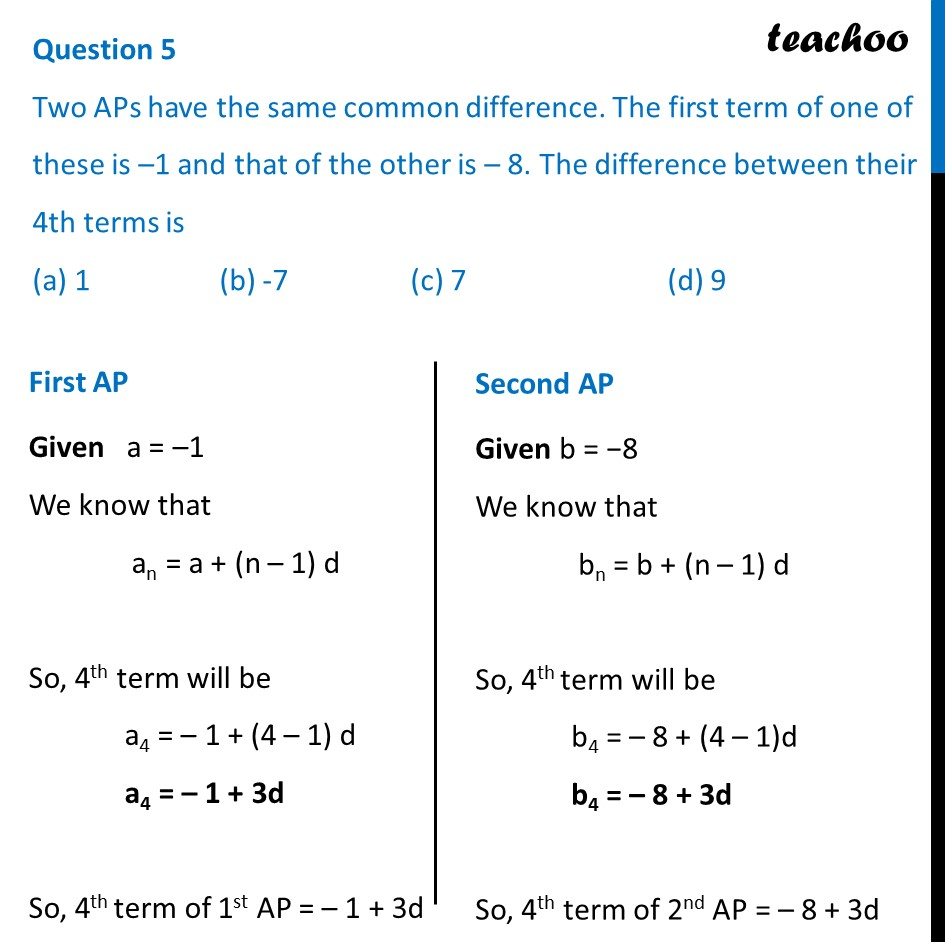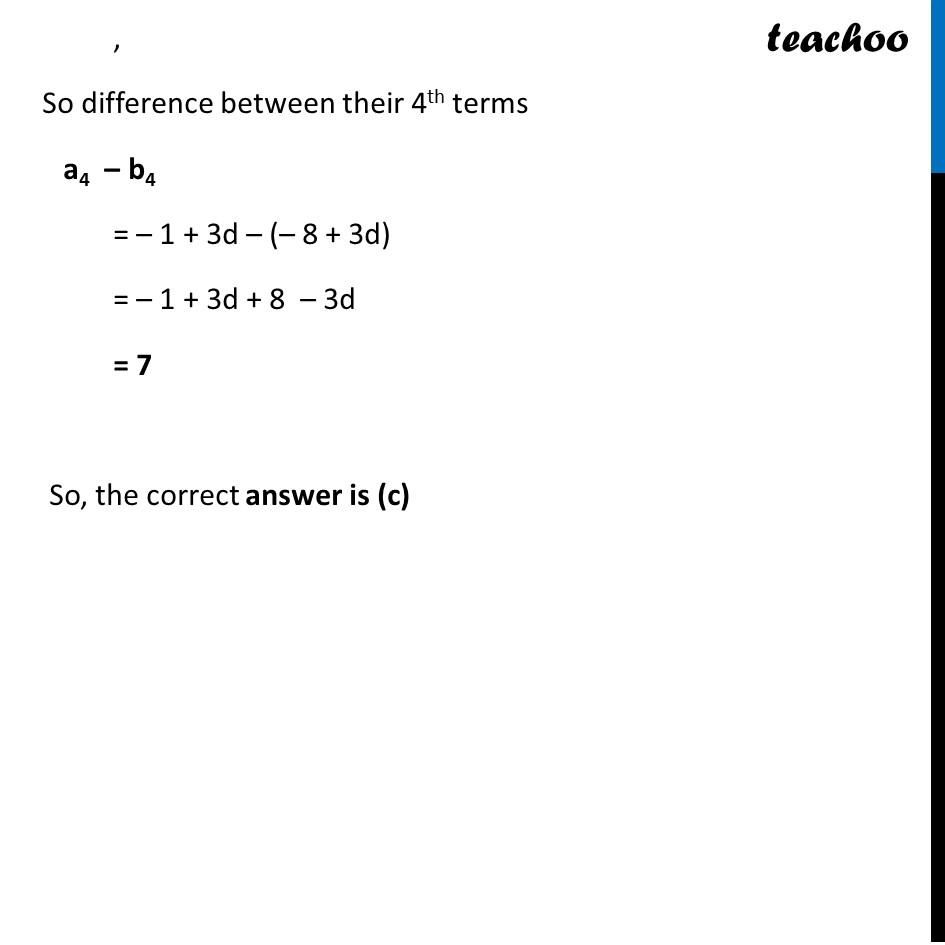CBSE Class 10 Sample Paper for 2024 Boards - Maths Standard

Class 10
Solutions of Sample Papers for Class 10 Boards

## (a) 1                  (b) -7                 (c) 7                    (d) 9

This question is similar to Ex-5.2, 12-Chapter 5 Class 10 Arithmetic ProgressionsLearn in your speed, with individual attention - Teachoo Maths 1-on-1 Class

### Transcript

First AP Given a = –1 We know that an = a + (n – 1) d So, 4th term will be a4 = – 1 + (4 – 1) d a4 = – 1 + 3d So, 4th term of 1st AP = – 1 + 3d Second AP Given b = −8 We know that bn = b + (n – 1) d So, 4th term will be b4 = – 8 + (4 – 1)d b4 = – 8 + 3d So, 4th term of 2nd AP = – 8 + 3d , So difference between their 4th terms a4 – b4 = – 1 + 3d – (– 8 + 3d) = – 1 + 3d + 8 – 3d = 7 So, the correct answer is (c)